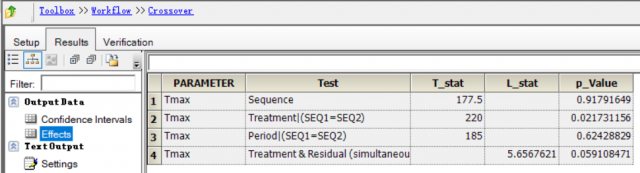## Question：Using Phoenix WinNonlin Crossover object for Tmax test [Nonparametrics]

Dear Helmut Schütz,

When using Phoenix WinNonlin Crossover object for Tmax testing, how can I judge whether it is equivalent by the result?I read the "The Use Of Non-Parametric Methods In The Statistical Analysis" document (PMID: 4556704), and I think he is doing the following steps to judge:

1. Determine if the P value of the sequence is greater than 0.05,
2. If the P value of the sequence is less than 0.05, the test of "Treatment" cannot be performed.
3. If the P value of the sequence is greater than 0.05, it is judged whether the P value of the treatment is greater than 0.05.
4. If the P value of the Treatment is greater than the equivalent, otherwise it is not equivalent.

5. If the P value of the sequence and the P value of the Period are both greater than 0.05, a sign test can be used instead of the rank sum test used in the above steps.

Assuming α = 0.05, all of the above P values are the results in the "Effects" table.

Is the above judgment process correct?If not, what is the correct judgment process?PARAMETER  Test                                T_stat    L_stat   p_Value Tmax       Sequence                            177.5              0.91791649 Tmax       Treatment|(SEQ1=SEQ2)                 220             0.021731156 Tmax       Period|(SEQ1=SEQ2)                    185              0.62428829 Tmax       Treatment & Residual (simultaneous)        5.6567621  0.059108471Best,

0521Ing. Helmut Schütz